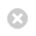Close

UCL Department of Biochemical Engineering

HomeMathematic Modelling and Analysis II

 Course Code BENG206P Level Two Credits 0.5 Module Tutor Dr Alex Kiparissides Assessment Three-hour written examination: 80% Coursework: 20% Prerequisites ENGS103P

Aims

To provide the core mathematics, modelling and analysis skills that underpin studies in engineering through a blended learning and teaching programme of mathematics that:
•    Emphasises the modelling of engineering systems and the analysis of data;
•    Integrates mathematics theory and engineering practice.

Learning Hours

Includes:

Lectures: 20h
Seminars/tutorials: 20h

Syllabus

By the end of the course students should be able to :

• Recognise the connections between mathematics and engineering, and how mathematical ideas are embedded in engineering contexts;
• Represent real-world systems from engineering in a mathematical framework;
• Identify and draw upon a range of advanced mathematical concepts, including Vector Calculus, Matrices and Eigenvectors/Values, Statistics, Series and Transforms, and Partial Differential Equations (PDEs) to analyse specific problems and identify the appropriate mathematics to realise a solution;
• Employ appropriate computer programming and modelling techniques and statistical analysis to efficiently solve and evaluate the performance of engineering systems;
• Relate the behaviour of the output of mathematical models to the underlying physical or conceptual models of interest;
• Carry our engineering problem solving both collaboratively in a team and independently;
• Present and interpret mathematical results in effective and appropriate ways to varied audiences, including non-mathematical engineering audiences.